Previous Page | Next Page

 The PHREG Procedure

## Example 64.12 Model Assessment Using Cumulative Sums of Martingale Residuals

The Mayo liver disease example of Lin, Wei, and Ying (1993) is reproduced here to illustrate the checking of the functional form of a covariate and the assessment of the proportional hazards assumption. The data represent 418 patients with primary biliary cirrhosis (PBC), among whom 161 had died as of the date of data listing. A subset of the variables is saved in the SAS data set Liver. The data set contains the following variables:

• Time, follow-up time, in years

• Status, event indicator with value 1 for death time and value 0 for censored time

• Age, age in years from birth to study registration

• Albumin, serum albumin level, in gm/dl

• Bilirubin, serum bilirubin level, in mg/dl

• Edema, edema presence

• Protime, prothrombin time, in seconds

The following statements create the data set Liver:

```data Liver;
input Time Status Age Albumin Bilirubin Edema Protime @@;
label Time="Follow-up Time in Years";
Time= Time / 365.25;
datalines;
400 1 58.7652 2.60 14.5 1.0 12.2 4500 0 56.4463 4.14  1.1 0.0 10.6
1012 1 70.0726 3.48  1.4 0.5 12.0 1925 1 54.7406 2.54  1.8 0.5 10.3
1504 0 38.1054 3.53  3.4 0.0 10.9 2503 1 66.2587 3.98  0.8 0.0 11.0
1832 0 55.5346 4.09  1.0 0.0  9.7 2466 1 53.0568 4.00  0.3 0.0 11.0
2400 1 42.5079 3.08  3.2 0.0 11.0   51 1 70.5599 2.74 12.6 1.0 11.5
3762 1 53.7139 4.16  1.4 0.0 12.0  304 1 59.1376 3.52  3.6 0.0 13.6
3577 0 45.6893 3.85  0.7 0.0 10.6 1217 1 56.2218 2.27  0.8 1.0 11.0

... more lines ...

1103 0 39.0000 3.83  0.9 0.0 11.2 1055 0 57.0000 3.42  1.6 0.0  9.9
691 0 58.0000 3.75  0.8 0.0 10.4  976 0 53.0000 3.29  0.7 0.0 10.6
;
run;
```

Consider fitting a Cox model for the survival time of the PCB patients with the covariates Bilirubin, log(Protime), log(Albumin), Age, and Edema. The log transform, which is often applied to blood chemistry measurements, is deliberately not employed for Bilirubin. It is of interest to assess the functional form of the variable Bilirubin in the Cox model. The specifications are as follows:

```ods graphics on;
proc phreg data=Liver;
model Time*Status(0)=Bilirubin logProtime logAlbumin Age Edema;
logProtime=log(Protime);
logAlbumin=log(Albumin);
assess var=(Bilirubin) /  resample seed=7548;
run;
ods graphics off;
```

The ASSESS statement creates a plot of the cumulative martingale residuals against the values of the covariate Bilirubin, which is specified in the VAR= option. The RESAMPLE option computes the-value of a Kolmogorov-type supremum test based on a sample of 1,000 simulated residual patterns.

Parameter estimates of the model fit are shown in Output 64.12.1. The plot in Output 64.12.2 displays the observed cumulative martingale residual process for Bilirubin together with 20 simulated realizations from the null distribution. This graphical display is requested by specifying the ODS GRAPHICS ON statement and the ASSESS statement. It is obvious that the observed process is atypical compared to the simulated realizations. Also, none of the 1,000 simulated realizations has an absolute maximum exceeding that of the observed cumulative martingale residual process. Both the graphical and numerical results indicate that a transform is deemed necessary for Bilirubin in the model.

Output 64.12.1 Cox Model with Bilirubin as a Covariate
 Wei-Lin-Weissfeld Model

The PHREG Procedure

Analysis of Maximum Likelihood Estimates
Parameter DF Parameter
Estimate
Standard
Error
Chi-Square Pr > ChiSq Hazard
Ratio
Bilirubin 1 0.11733 0.01298 81.7567 <.0001 1.124
logProtime 1 2.77581 0.71482 15.0794 0.0001 16.052
logAlbumin 1 -3.17195 0.62945 25.3939 <.0001 0.042
Age 1 0.03779 0.00805 22.0288 <.0001 1.039
Edema 1 0.84772 0.28125 9.0850 0.0026 2.334

Output 64.12.2 Cumulative Martingale Residuals vs Bilirubin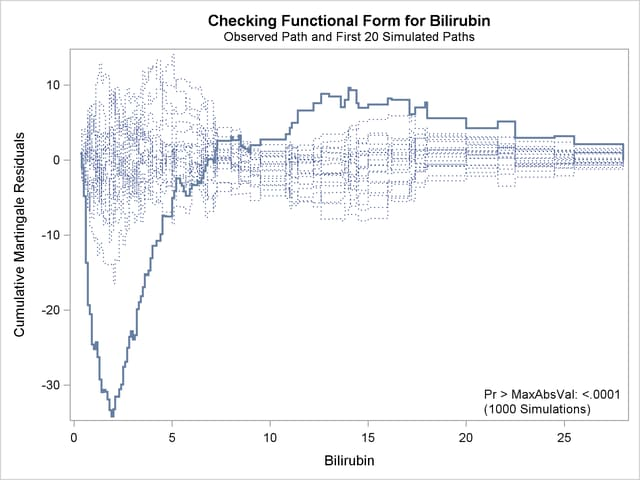The cumulative martingale residual plots in Output 64.12.3 provide guidance in suggesting a more appropriate functional form for a covariate. The four curves were created from simple forms of misspecification by using 1,000 simulated times from a exponential model with 20% censoring. The true and fitted models are shown in Table 64.13. The following statements produce Output 64.12.3.

```data sim(drop=tmp);
p = 1 / 91;
seed = 1;
do n = 1 to 10000;
x1 = rantbl( seed, p, p, p, p, p, p, p, p, p, p,
p, p, p, p, p, p, p, p, p, p,
p, p, p, p, p, p, p, p, p, p,
p, p, p, p, p, p, p, p, p, p,
p, p, p, p, p, p, p, p, p, p,
p, p, p, p, p, p, p, p, p, p,
p, p, p, p, p, p, p, p, p, p,
p, p, p, p, p, p, p, p, p, p,
p, p, p, p, p, p, p, p, p, p );

x1 = 1 + ( x1 - 1 ) / 10;
x2= x1 * x1;
x3= x1 * x2;
status= rantbl(seed, .8);
tmp= log(1-ranuni(seed));
t1= -exp(-log(x1)) * tmp;
t2= -exp(-.1*(x1+x2)) * tmp;
t3= -exp(-.01*(x1+x2+x3)) * tmp;
tt= -exp(-(x1>5)) * tmp;
output;
end;
run;

proc sort data=sim;
by x1;
run;

proc phreg data=sim noprint;
model t1*status(2)=x1;
output out=out1 resmart=resmart;
run;

proc phreg data=sim noprint;
model t2*status(2)=x1;
output out=out2 resmart=resmart;
run;

proc phreg data=sim noprint;
model t3*status(2)=x1 x2;
output out=out3 resmart=resmart;
run;

proc phreg data=sim noprint;
model tt*status(2)=x1;
output out=out4 resmart=resmart;
run;

data out1(keep=x1 cresid1);
retain cresid1 0;
set out1;
by x1;
cresid1 + resmart;
if last.x1  then output;
run;

data out2(keep=x1 cresid2);
retain cresid2 0;
set out2;
by x1;
cresid2 + resmart;
if last.x1  then output;
run;

data out3(keep=x1 cresid3);
retain cresid3 0;
set out3;
by x1;
cresid3 + resmart;
if last.x1  then output;
run;

data out4(keep=x1 cresid4);
retain cresid4 0;
set out4;
by x1;
cresid4 + resmart;
if last.x1  then output;
run;

data all;
set out1;
set out2;
set out3;
set out4;
run;

proc template;
define statgraph MisSpecification;
BeginGraph;
entrytitle "Covariate Misspecification";
layout lattice / columns=2 rows=2 columndatarange=unionall;

columnaxes;
columnaxis / display=(ticks tickvalues label) label="x";
columnaxis / display=(ticks tickvalues label) label="x";
endcolumnaxes;

cell;
entry "(a) Data: log(X), Model: X";
layout overlay / xaxisopts=(display=none)
yaxisopts=(label="Cumulative Residual");
seriesplot y=cresid1 x=x1 / lineattrs=GraphFit;
endlayout;
endcell;

cell;
entry "(b) Data: X*X, Model: X";
layout overlay / xaxisopts=(display=none)
yaxisopts=(label=" ");
seriesplot y=cresid2 x=x1 / lineattrs=GraphFit;
endlayout;
endcell;

cell;
entry "(c) Data: X*X*X, Model: X*X";
layout overlay / xaxisopts=(display=none)
yaxisopts=(label="Cumulative Residual");
seriesplot y=cresid3 x=x1 / lineattrs=GraphFit;
endlayout;
endcell;

cell;
entry "(d) Data: I(X>5), Model: X";
layout overlay / xaxisopts=(display=none)
yaxisopts=(label=" ");
seriesplot y=cresid4 x=x1 / lineattrs=GraphFit;
endlayout;
endcell;

endlayout;
EndGraph;
end;

proc sgrender data=all template=MisSpecification;
run;
```

Output 64.12.3 Typical Cumulative Residual Plot Patterns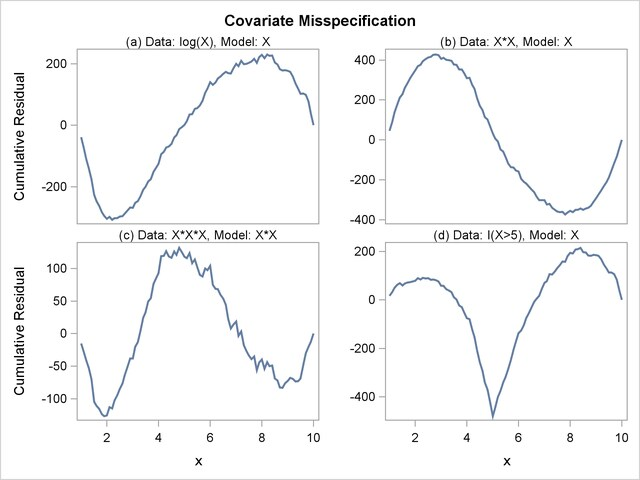Table 64.13 Model Misspecifications

Plot

Data

Fitted Model

(a)

log(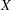)(b)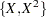(c)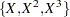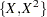(d)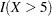The curve of observed cumulative martingale residuals in Output 64.12.2 most resembles the behavior of the curve in plot (a) of Output 64.12.3, indicating that log(Bilirubin) might be a more appropriate term in the model than Bilirubin.

Next, the analysis of the natural history of the PBC is repeated with log(Bilirubin) replacing Bilirubin, and the functional form of log(Bilirubin) is assessed. Also assessed is the proportional hazards assumption for the Cox model. The analysis is carried out by the following statements:

```ods graphics on;
proc phreg data=Liver;
model Time*Status(0)=logBilirubin logProtime logAlbumin Age Edema;
logBilirubin=log(Bilirubin);
logProtime=log(Protime);
logAlbumin=log(Albumin);
assess var=(logBilirubin) ph / crpanel resample seed=19;
run;
ods html close;
```

The SEED= option specifies a integer seed for generating random numbers. The CRPANEL option in the ASSESS statement requests a panel of four plots. Each plot displays the observed cumulative martingale residual process along with two simulated realizations. The PH option checks the proportional hazards assumption of the model by plotting the observed standardized score process with 20 simulated realizations for each covariate in the model.

Output 64.12.4 displays the parameter estimates of the fitted model. The cumulative martingale residual plots in Output 64.12.5 and Output 64.12.6 show that the observed martingale residual process is more typical of the simulated realizations. The-value for the Kolmogorov-type supremum test based on 1,000 simulations is 0.052, indicating that the log transform is a much improved functional form for Bilirubin.

Output 64.12.4 Model with log(Bilirubin) as a Covariate
 Wei-Lin-Weissfeld Model

The PHREG Procedure

Analysis of Maximum Likelihood Estimates
Parameter DF Parameter
Estimate
Standard
Error
Chi-Square Pr > ChiSq Hazard
Ratio
logBilirubin 1 0.87072 0.08263 111.0484 <.0001 2.389
logProtime 1 2.37789 0.76674 9.6181 0.0019 10.782
logAlbumin 1 -2.53264 0.64819 15.2664 <.0001 0.079
Age 1 0.03940 0.00765 26.5306 <.0001 1.040
Edema 1 0.85934 0.27114 10.0447 0.0015 2.362

Output 64.12.5 Panel Plot of Cumulative Martingale Residuals versus log(Bilirubin)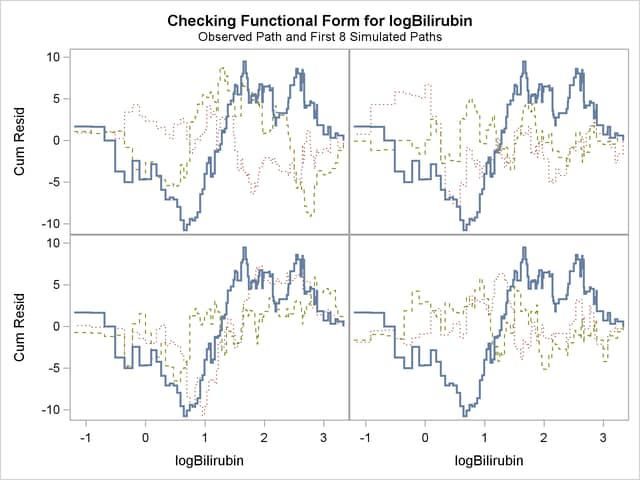Output 64.12.6 Cumulative Martingale Residuals versus log(Bilirubin)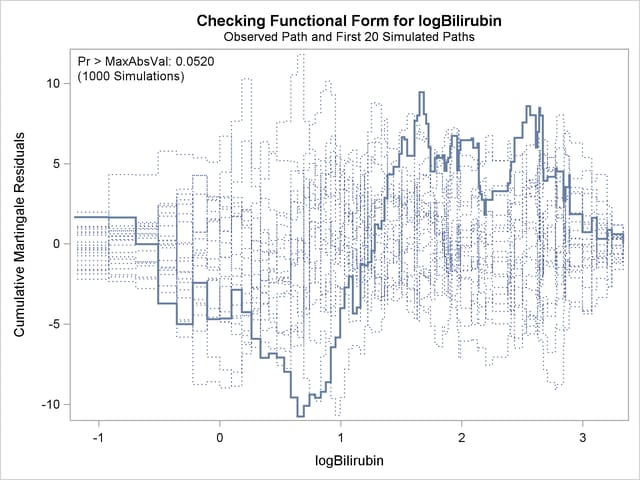Output 64.12.7 and Output 64.12.8 display the results of proportional hazards assumption assessment for log(Bilirubin) and log(Protime), respectively. The latter plot reveals nonproportional hazards for log(Protime).

Output 64.12.7 Standardized Score Process for log(Bilirubin)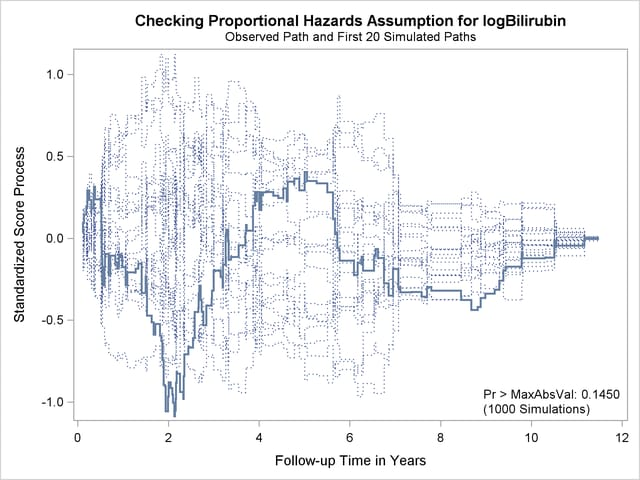[

Output 64.12.8 Standardized Score Process for log(Protime)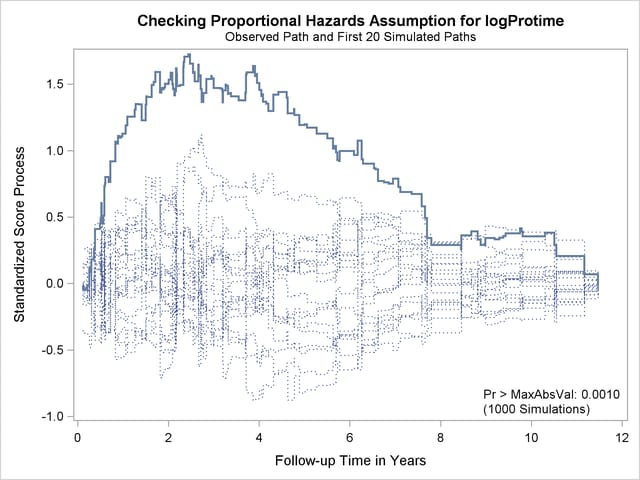Plots for log(Albumin), Age, and Edema are not shown here. The Kolmogorov-type supremum test results for all the covariates are shown in Output 64.12.9. In addition to log(Protime), the proportional hazards assumption appears to be violated for Edema.

Output 64.12.9 Kolmogorov-Type Supremum Tests for Proportional Hazards Assumption
Supremum Test for Proportionals Hazards Assumption
Variable Maximum Absolute
Value
Replications Seed Pr >
MaxAbsVal
logBilirubin 1.0880 1000 19 0.1450
logProtime 1.7243 1000 19 0.0010
logAlbumin 0.8443 1000 19 0.4330
Age 0.7387 1000 19 0.4620
Edema 1.4350 1000 19 0.0330Previous Page | Next Page | Top of Page